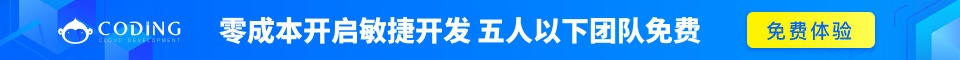# Ruby 范围（Range）

• 作为序列的范围
• 作为条件的范围
• 作为间隔的范围

## 作为序列的范围

Ruby 使用 ''..''''...'' 范围运算符创建这些序列。两点形式创建一个包含指定的最高值的范围，三点形式创建一个不包含指定的最高值的范围。

```(1..5)        #==> 1, 2, 3, 4, 5
(1...5)       #==> 1, 2, 3, 4
('a'..'d')    #==> 'a', 'b', 'c', 'd'
```

```#!/usr/bin/ruby

\$, =", "   # Array 值分隔符
range1 = (1..10).to_a
range2 = ('bar'..'bat').to_a

puts "#{range1}"
puts "#{range2}"
```

```1, 2, 3, 4, 5, 6, 7, 8, 9, 10
bar, bas, bat
```

```#!/usr/bin/ruby

# Assume a range
digits = 0..9

puts digits.include?(5)
ret = digits.min
puts "Min value is #{ret}"

ret = digits.max
puts "Max value is #{ret}"

ret = digits.reject {|i| i < 5 }
puts "Rejected values are #{ret}"

digits.each do |digit|
puts "In Loop #{digit}"
end
```

```true
Min value is 0
Max value is 9
Rejected values are 5, 6, 7, 8, 9
In Loop 0
In Loop 1
In Loop 2
In Loop 3
In Loop 4
In Loop 5
In Loop 6
In Loop 7
In Loop 8
In Loop 9
```

## 作为条件的范围

```while gets
print if /start/../end/
end
```

```#!/usr/bin/ruby

score = 70

result = case score
when 0..40: "Fail"
when 41..60: "Pass"
when 61..70: "Pass with Merit"
when 71..100: "Pass with Distinction"
else "Invalid Score"
end

puts result
```

```Pass with Merit
```

## 作为间隔的范围

```#!/usr/bin/ruby

if ((1..10) === 5)
puts "5 lies in (1..10)"
end

if (('a'..'j') === 'c')
puts "c lies in ('a'..'j')"
end

if (('a'..'j') === 'z')
puts "z lies in ('a'..'j')"
end
```

```5 lies in (1..10)
c lies in ('a'..'j')
```